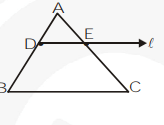# Using Theorem 6.1,

Question.

Using Theorem 6.1, prove that a line drawn through the mid-point of one side of a triangle parallel to another side bisects the third side.

Solution:

In $\triangle \mathrm{ABC}, \mathrm{D}$ is mid point of $\mathrm{AB}$ (see figure)i.e., $\frac{A D}{D B}=1$

Straight line $\ell \| \mathrm{BC}$.

Line $\ell$ is drawn through $\mathrm{D}$ and it meets $\mathrm{AC}$ at $\mathrm{E}$.

By Basic Proportionality Theorem

$\frac{A D}{D B}=\frac{A E}{E C} \Rightarrow \frac{A E}{E C}=1[F r o m(1)]$

$\Rightarrow \mathrm{AE}=\mathrm{EC} \Rightarrow \mathrm{E}$ is mid point of $\mathrm{AC}$.